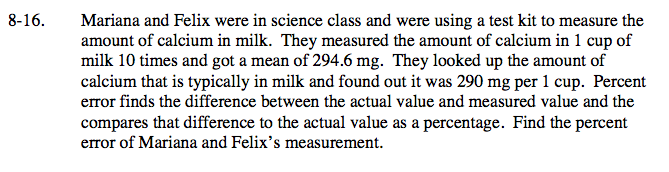Home > ACC7 > Chapter cc28 > Lesson cc28.1.1 > Problem8-16

8-16.

Mariana and Felix were in science class and were using a test kit to measure the amount of calcium in milk. They measured the amount of calcium in 1 cup of milk 10 times and got a mean of 294.6 mg. They looked up the amount of calcium that is typically in milk and found out it was 290 mg per 1 cup. Percent error finds the difference between the actual value and measured value and the compares that difference to the actual value as a percentage. Find the percent error of Mariana and Felix’s measurement. Homework Help ✎Find the difference between the measured value and the actual value.
294.6 − 290 = 4.6

Divide the difference by the actual value.

$\frac{4.6}{290}=0.0158$

Multiply this number by 100% to get the percent error.
(0.0158)(100%) = about 1.6%